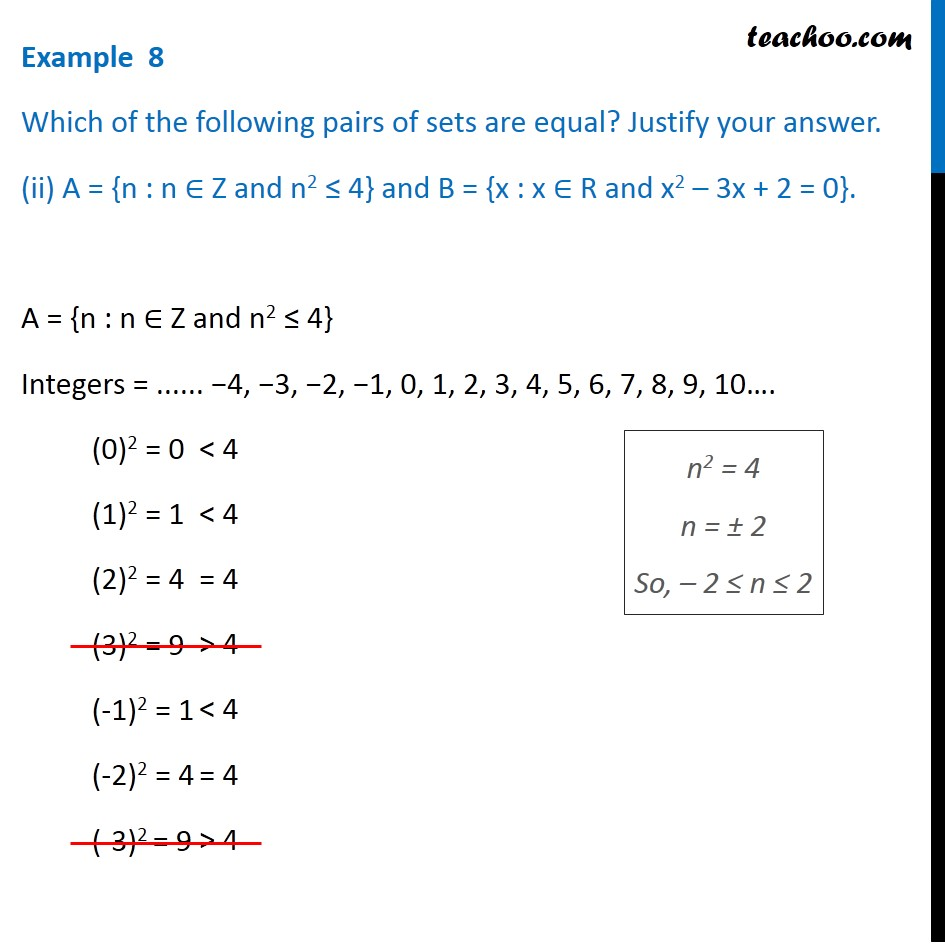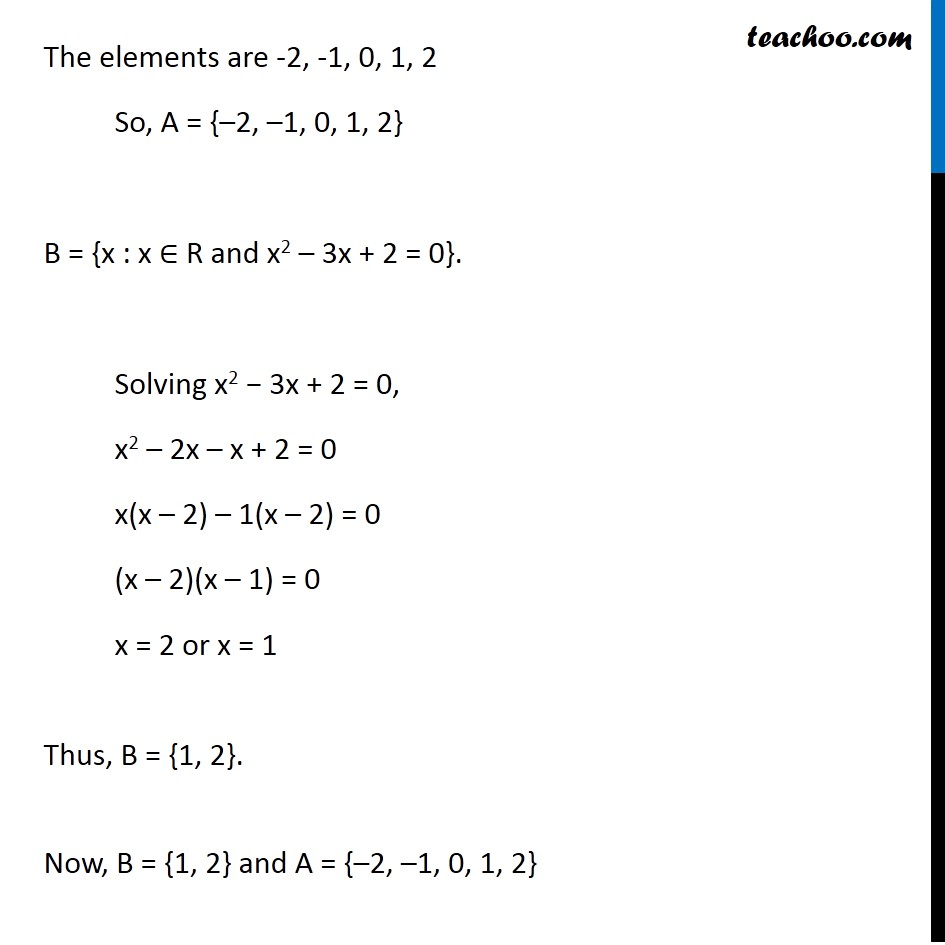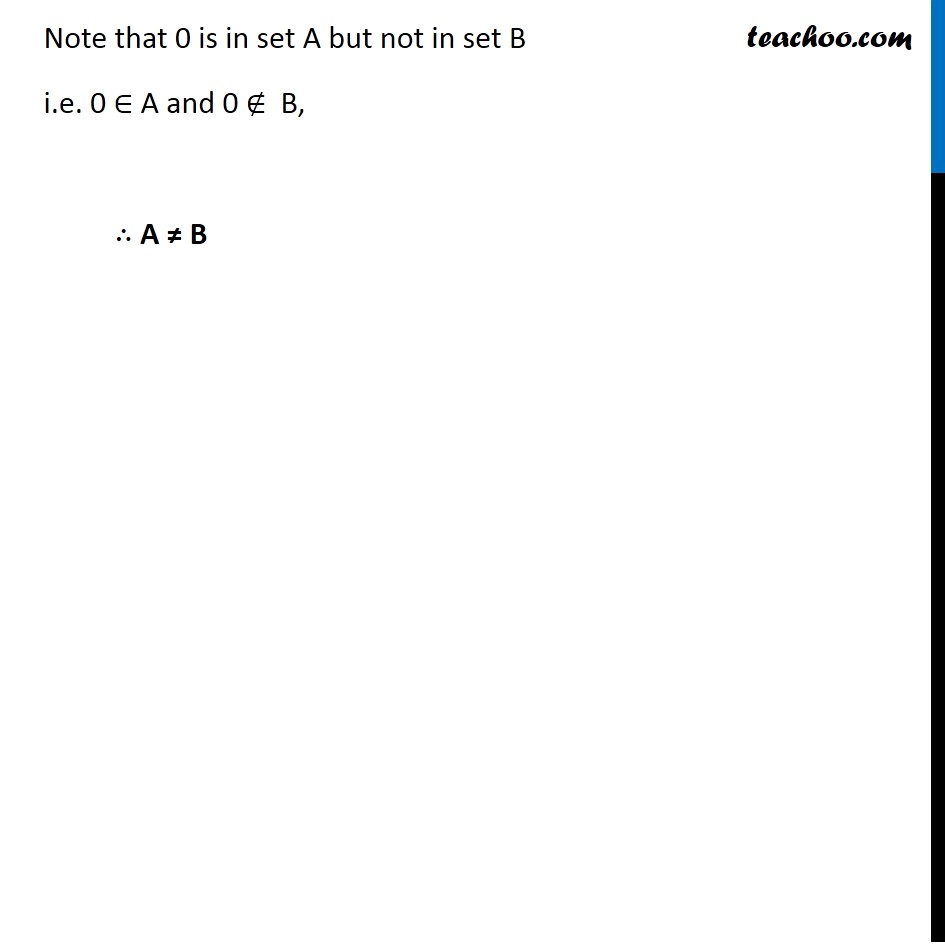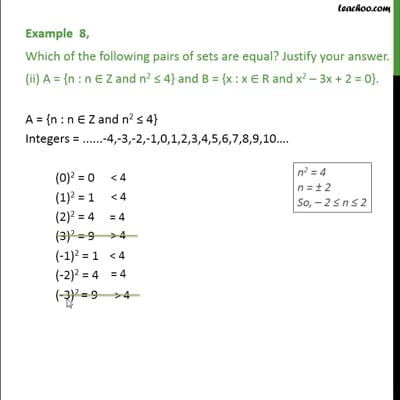Examples

Chapter 1 Class 11 Sets
Serial order wiseThis video is only available for Teachoo black users

Maths Crash Course - Live lectures + all videos + Real time Doubt solving!

### Transcript

Example 8 Which of the following pairs of sets are equal? Justify your answer. (ii) A = {n : n ∈ Z and n2 ≤ 4} and B = {x : x ∈ R and x2 – 3x + 2 = 0}. A = {n : n ∈ Z and n2 ≤ 4} Integers = ...... −4, −3, −2, −1, 0, 1, 2, 3, 4, 5, 6, 7, 8, 9, 10…. (0)2 = 0 (1)2 = 1 (2)2 = 4 (3)2 = 9 (-1)2 = 1 (-2)2 = 4 (-3)2 = 9 The elements are -2,-1,0,1,2, So, A = {–2, –1, 0, 1, 2} B = {x : x ∈ R and x2 – 3x + 2 = 0}. Solving x2 - 3x + 2 = 0, x2 – 2x – x + 2 = 0 x(x – 2) – 1(x – 2) = 0 (x – 2)(x – 1) = 0 x = 2 or x = 1 Thus, B = {1, 2}. Now, B = {1, 2} and A = {–2, –1, 0, 1, 2} Note that 0 is in set A but not in set B i.e. 0 ∈ A and 0 ∉ B, ∴ A ≠ B